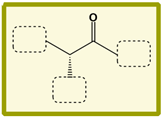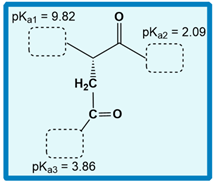## Biochemistry

Learn the toughest concepts covered in Biochemistry with step-by-step video tutorials and practice problems by world-class tutors

3. Amino Acids

# Zwitterion

1
concept

## Zwitterion2m
Play a video:
So now that we've talked about the groupings for all 20 amino acids and each of their individual are groups, we're now gonna talk about sweater ions. So it turns out that the common backbone for all 20 amino acids is predominantly as Witter ion at physiological pH. And recall that physiological pH is right around a ph of about seven. And recall also that when we say predominant structure, what we really mean is that this structure is in the highest concentration amongst all the other types of structure. And so this fact here is actually gonna become a common known fact. Uh, that's gonna be part of our basic knowledge of amino acids, even though it might be new information to you right now. Now it turns out that as Witter ion so the definition of his Witter ion is just a die polar molecule that bears or that has two groups that air opposite, Lee charged. So two groups of opposite charge and the charges on those two groups are a result of acid base reactions, or H plus transfers or proton transfers. And so, in our example, noticed that we have the common structure for all 20 amino acids. So we have our Alfa Carbon and the center here we have our central hydrogen. We have our car box late group on the right and our amino group on the left and then down below. We have our our group. And so which will also notice is that we have the two groups bearing charges highlighted. So on the left, here we have our amino group, which notice has a positive charge on it. And then on the right, we have our car, Boxley and I am, which has a negative charge on this oxygen shown there. And so because there are two groups that have opposite charged on the same molecule, this is a sweeter ion and the backbone of all 20 amino acids isas Witter ion, specifically at physiological pH. Which is right around Ph of seven. And so this is again gonna be common knowledge. That is gonna be very basic knowledge that we're gonna be referring to throughout our course. So it's good Thio get this introduced. And so we'll be able to get a little bit of practice utilizing this concept in our next practice video. So I'll see you guys there
2
Problem

Which shows the proper structure of Leu at physiological pH?

3
concept

## Zwitterion8m
Play a video:
4
example

## Zwitterion Example 22m
Play a video:
Alright. So here's an example problem that says at Ph. Two, which is the predominant structure of failing, and we're giving these two p. K S P. K one, which is 9.62 and P k two, which is 2.32 And so we know from our previous lesson videos that veiling is a non polar amino acid with a V shaped our group. And so, looking at all of these are groups down below in our answer options, which are highlighted in blue notice that they all have the same structure and the same V shape. So they all have the correct our group for veiling, and we're going to need to determine the correct answer by determining the predominant structure off Valeant's backbone at a ph of two. And so recall from our previous lesson videos that Alfa Amino groups have. P K is in the ballpark range of about 9 to 10.5, and so PK one here must be the PKK of the Amino Group and also recall that Alfa Car Boxer groups have PK is in the ballpark range of about two and so p k two here must be for the car Boxer group. And we know that we can determine the predominant structure by independently comparing these two p. K s to the ph of the solution. And so we can start with the amino group PK. And so we know that a pH of to is less than the amino group P K, which is equal to 9.62 And so we know from our previous lesson videos that when the pH is less than the peak A that the conjugate acid form predominates and the conjugate acid is a protein ated form and the protein ated form of the amino group is going to be an NH three plus. And so we can eliminate any answer option that does not have an NH three plus for the Amino Group. And so this one notice has an N h two plus, so we can cross that off. And this one just has an N h two so we can cross that off a swell and notice that both option A and D have the correct NH three plus form. And so we're gonna need to look at the car Boxer group next, and so we know that a pH of two is also less than the car boxful PK of two point 32 And so again, when the pH is less than the PK, the conjugate acid form predominates, and the conjugate acid form is protein ated. So we expect our car box a group to be protein ated and so noticed that the car box a group for option A is not protein ated so we could go ahead and cross that off and looking at option D notice that it is protein ated. And so that means that d here is gonna be the correct answer. And that concludes this practice or this example problem. So we'll be able to get some practice in our next couple of videos, and I'll see you guys there.
5
Problem

Fill in the groups for the predominant structure of Ala at pH 13?6
Problem

Fill in the appropriate groups for Asp at pH 4.3.7
Problem

Draw the predominant structure of Arg at pH 6.5? (pK a1 = 9.04, pKa2 = 2.17, pKa3 = 12.48).